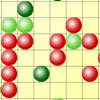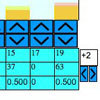You may also likeSpinners

How do scores on dice and factors of polynomials relate to each other?Epidemic Modelling

Use the computer to model an epidemic. Try out public health policies to control the spread of the epidemic, to minimise the number of sick days and deaths.Distribution Maker

This tool allows you to create custom-specified random numbers, such as the total on three dice.

Random Squares

Why do this problem?

This is a very open-ended task in which the learner is encouraged to contemplate the notion of randomness. Of course, although every individual pattern has just as much chance of emerging as any other individual pattern, there are many patterns which are qualitatively 'similar' to each other. Attempting to quantify this 'similarity' is a good exercise in mathematical thinking.

Possible approach

In discussion, ideas will emerge about the nature of randomness. Be sure to note that students are all aware that all patterns are equally likely. It can be used productively at any point during the study of statistics.

Key questions

• What can we see?
• What similarities and what differences occur?
• Do any patterns look to the eye less likely than others? Why?

Possible extension

Various further calculations concerning the squares can be suggested. In particular, how might one estimate the probability that an individual square is red given a fully coloured grid? How might we devise a confidence interval for this estimate?

Possible support

Try the Can't find a coin? problem first